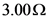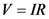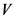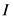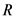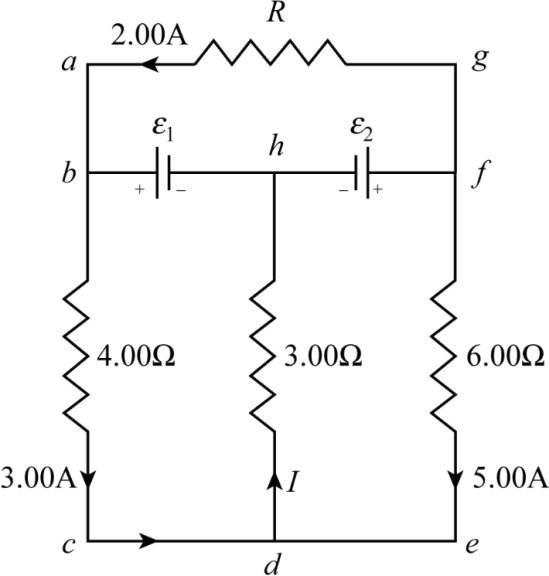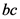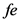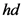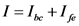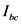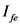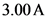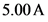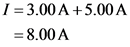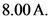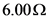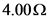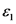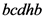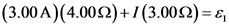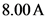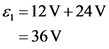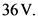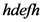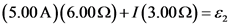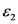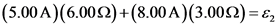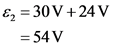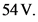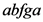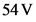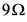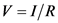# Find the current in the 3.00 ω resistor. (note that three currents are given.)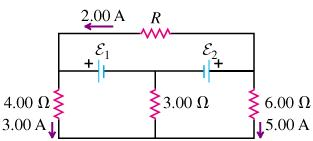26.23) 1.Find the current in the 3.00 resistor. (Note that three
currents are given.)2.Find the unknown emfs and .3.Find the resistance .General guidance

Concepts and reason
The concepts required to solve the problem are Ohm’s law and Kirchhoff’s junction rule.
First, from the given currents, find the current in theresistor. Using Kirchhoff’s voltage law, find the unknown emfs. Similarly, using the Kirchhoff’s voltage law, find the unknown resistance.

Fundamentals

Ohm’s law states that current between two points in a circuit is proportional to the potential difference between the points. The constant of proportionality is called the resistance.

Here, is the potential difference, is the current andis the resistance.
Kirchhoff’s circuit laws for current and voltage are applied in electrical circuits. Kirchhoff’s current law states that the total current entering a nod is equal to the total current leaving the node.
Kirchhoff’s voltage law states that the total voltage around a closed loop is zero.

Step-by-step

Step 1 of 4

(26.23.1)
The below figure shows the direction of current flowing through the circuit.

The current in the lineand in the linecombine and flow together through the lineas current.
So, the current in the lineis given by

Here, is the current in the lineandis the current in the line.
Substituteforandforto find the current.

Part 26.23.1
The current in the is

The current through theresistor is the sum of the current through theresistor and through theresistor.

The incorrect view of Kirchhoff’s current law is that “ The sum of current entering the junction is not equal to the sum of the current leaving the junction” but the correct view is that “ The sum of current entering the junction is equal to the sum of the current leaving the junction”

Using Kirchhoff’s junction rule, find the unknown emf.

Step 2 of 4

(26.23.2.1)
Applying Kirchhoff’s voltage law in loop,

Substituteforto find the emf.

So, the emfis

Part 26.23.2.1
The emfis

According to Kirchhoff’s second law, the voltage at a closed loop is zero. Applying this law in the loop, the emfis the sum of the products of the current and resistor in wire and the current and resistor in wire.

The incorrect view for Kirchoff’s voltage law is that if the potential source is connected in series with negative of one is connected to positive of other source then the potential cannot be added. But the correct view is that the potential can be added.

Using Kirchhoff’s junction rule, find the unknown emf.

Step 3 of 4

(26.23.2.2)
Applying Kirchhoff’s voltage law in loop,

Substituteforto find the emf.

So, the emfis

Part 26.23.2.2
The emfis

Applying Kirchhoff’s second law in the loop, the emfis the sum of the products of the current and resistor in wire and the current and resistor in wire.

A wrong way to define the junction rule is to say that the net current in the loop is zero. The correct statement would be that the net current at a junction is zero.

Using Kirchhoff’s junction rule, find the resistance.

Step 4 of 4

(26.23.3)
Applying Kirchhoff’s voltage law in loop,

Substituteforand forto find the resistance.

Part 26.23.3
The resistanceis.

Applying Kirchhoff’s second law in the loop, the resistanceis the sum emfsand.

According to Ohm’s law, the voltage is not.
The correct equation is.

Part 26.23.1
The current in the is

Part 26.23.2.1
The emfis

Part 26.23.2.2
The emfis

Part 26.23.3
The resistanceis.

Part 26.23.1
The current in the is

Part 26.23.2.1
The emfis

Part 26.23.2.2
The emfis

Part 26.23.3
The resistanceis.

3.00Q
V IR
R
3.00Q
R 2.00A ి E1 h f b 4.00 3.002 6.00S 3.00AV 5.00A с e d కా
bc
fe
hd
hd
be fe
be
bc
fe
3.00 A
be
5.00 A
I = 3.00 A +5.00 A =8.00 A
3.00Q
6.00
4.00 2
bcdhb
|(3.00 A) (4.00)1 (3.002)= e
8.00A
(3.00 A) (4.00)+(8.00 A) (3.002)= c,
& 12V+24V =36V
bcdhb
bc
hd
&2
hdefh
|(5.00 A) (6.00s2)+1 (3.00 2)=E,
8.00A
&2
(5.00 A) (6.00)+(8.00 A)(3.00) c, =
&2
30V 24V E =54V
hdefh
&2
hd
fe
R
abfga
&2-6( (2A)R R=-& 2A
36 V
54 V
&2
R
54V -36 V R = 2A 18V 2A =90
abfga
R
&2
= 1 /R V
V IR
3.00Q
8.00 A
3.00Q
8.00 A
3.00Q
5.00 A
3.00Q
3.00 А.
3.00Q
2.00 A
36V
36V
We were unable to transcribe this image